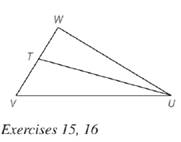Chapter 5.6, Problem 16E### Elementary Geometry for College St...

6th Edition
Daniel C. Alexander + 1 other
ISBN: 9781285195698

#### Solutions

Chapter
Section### Elementary Geometry for College St...

6th Edition
Daniel C. Alexander + 1 other
ISBN: 9781285195698
Textbook Problem
3 views

# Given: U T → bisects ∠ W U V , W U = 9 , U V = 12 , W V = 9 Find: W TTo determine

To find:

WT.

Explanation

Given:

UT bisects WUV, WU=9,UV=12,WV=9.

Theorem used:

Angle bisector theorem:

If a ray bisects one angle of a triangle, then it divides the opposite side into segments whose lengths are proportional to the lengths of the two sides that form the bisected angle.

Calculation:

Given: UT bisects WUV.

Then by angle-bisector theorem, the ray UT divides the opposite side into segments whose lengths are proportional to the lengths of the two sides that form the bisected angle.

TVWT=UVWU

We know that if ab=cd then a+bb=c+dd.

TV+WTWT=UV+WUWU

From the figure, we see that, TV+WT=WV

### Still sussing out bartleby?

Check out a sample textbook solution.

See a sample solution

#### The Solution to Your Study Problems

Bartleby provides explanations to thousands of textbook problems written by our experts, many with advanced degrees!

Get Started